## Quiz 11 : Handling Project UncertaintyLooking for Economics Homework Help?# Quiz 11 : Handling Project Uncertainty

Given information: • Machine cost is \$25,000. • Annual operating cost is \$3,000. • Salvage value at the end of 6 years is \$5,000. Formula: Annual saving: Annual saving can be calculated by using the following formula:Where, P = Present worth A = Annual equivalent cash flow i = Interest rate N = Number of years a.Annual savings in labor: Investor desired for 10 percent return from the investment. Annual saving in labor can be calculated by using the equation (1):…… (1)Present worth of investment: Equation (1) is used to convert the present worth of investment into annual cash flow.Hence, to obtain 10 percent return on investment, the savings in labor from this machine should be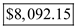or more. b.Change in useful life: Useful life of the machine is 5 years. Equation (1) is used to convert the present worth of investment into annual cash flow.Hence, to obtain 10 percent return on investment, the savings in labor from this machine should be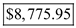or more. c.Increase in annual operating cost: Since, the annual operating cost increased by 10 percent, the new annual operating cost is \$3,300. By using of equation (1), the value of annual saving can be calculated as follows:Hence, to obtain 10 percent return on investment, the savings in labor from this machine should be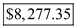or more than it.

Sensitivity analysis of present worth (PW): Present worth of an investment is as follows: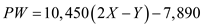…… (1)Sales price, X is \$20 and variable production costs, Y is \$10 respectively. Substitute the respective values in Equation (1) to obtain the present worth of the investment.Hence, the present worth of the investment is \$305,610. Sales price increased by 10 percent; hence, the new sales price is 22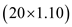. Substitute the respective values in Equation (1) to obtain the present worth of investment at new price.Hence, the present worth of the investment at new price is \$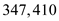. Change in present worth as 10 percent increase in sales price is calculated as follows:The present worth increased by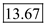percent; hence, option c is correct.

Given information: • Price of the truck is \$75,000. • Other cost is \$15,000. Thus, total cost is \$90,000. • Salvage value after 5 years is \$20,000. • Annual saving is \$35,000. • Depreciated at 5 year MACRS class • Marginal tax rate is 40%. • MARR rate is 15%. a.Profit from the investment: Table-1 shows the recovery rate under General Depreciation System for 5 years. Table-1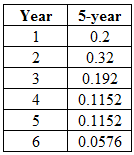Depreciation: Depreciation can be calculated by using the following formula.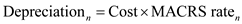…… (1)First year depreciation: Substitute the respective values in equation (1) to calculate the deprecation in year 1.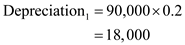Depreciation in the first year is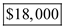. Taxable income: Taxable income can be calculated by using the following formula.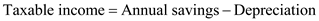…… (2)First year taxable income: Substitute the respective values in equation (2) to calculate the taxable income in year 1.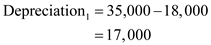Depreciation in the first year is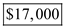. Tax payment: Tax payment can be calculated by using the following formula.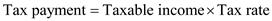…… (3)First year tax payment: Substitute the respective values in equation (3) to calculate the tax payment in year 1.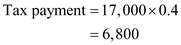First year tax payment is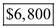. After tax cash flow: After tax cash flow (ATCF) can be calculated by using the following formula.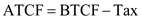…… (4)First year ATCF: Substitute the respective values in Equation (4) to calculate the first year ATCf.First year ATCF is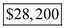. Taxable gain: Taxable gain can be calculated by using the following formula. When the asset is disposed, half year depreciation should be calculated.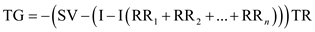…… (4.a)Where, TG = taxable gain TR = tax rate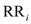=recovery rate of i th year SV = salvage value I = initial cost Since the machine sold before the life time, the final year MACRS value should be consider the 50%. Substitute the respective values in Equation (4.a) to calculate the taxable gain.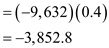Taxable gain is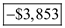. After tax cash flow: Fifth year half of the depreciation should be calculated. Table-1 shows the after tax cash flow of the project that obtained by using Equation (1), (2), (3) and (4). Table-2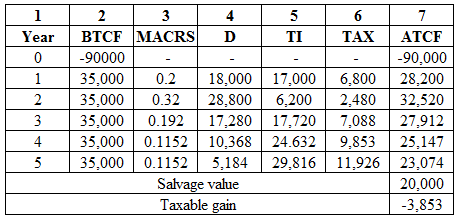Present equivalent worth (P): Present equivalent worth of future value can be calculated as follows: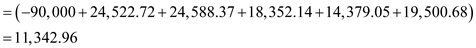Since the present worth of the truck is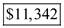, the project is economically feasible. b.After tax cash flow: Fifth year half of the depreciation should be calculated. Table-1 shows the after tax cash flow of the project that obtained by using Equation (1), (2), (3) and (4). Table-3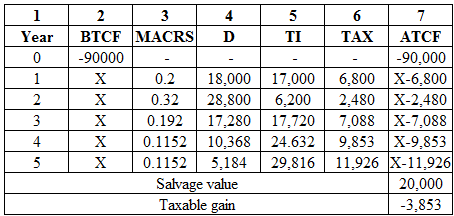Present equivalent worth (P): Present equivalent worth of future value can be calculated by suing the following formula:…… (5)Substitute the respective values in equation (5) and assume the value of X as \$35,000 by trial and error method. The present value should be equal to \$0.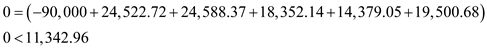The present worth of the truck is, Since the present worth is greater than zero, decrease the value of X to \$34,930.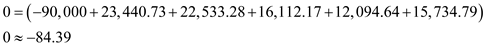Since the present worth is nearly zero, the value of X is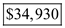. c.After tax cash flow: Fifth year half of the depreciation should be calculated. Table-1 shows the after tax cash flow of the project that obtained by using Equation (1), (2), (3) and (4). Table -4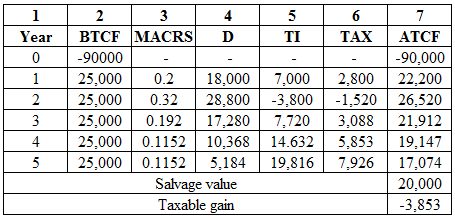Present equivalent worth (P): Present equivalent worth of future value can be calculated as follows: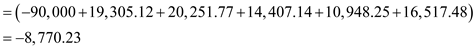The present worth of the truck is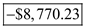. Since the project's present worth is negative, it is economically not feasible.

There is no answer for this question

There is no answer for this question

There is no answer for this question

There is no answer for this question

There is no answer for this question

There is no answer for this question

There is no answer for this question

There is no answer for this question

There is no answer for this question

There is no answer for this question